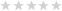Customer Service
Live Chat

Information

Advanced Engineering Mathematics 9th Edition by Erwin Kreyszig

Product Code: 0471728977
Availability: In Stock
Price:
₦3,400.00

Qty: - +
- OR -0 reviews  |  Write a review
2008           0471728977      9

This market leading text is known for its comprehensive coverage, careful and correct mathematics, outstanding exercises and self contained subject matter parts for maximum flexibility.

Thoroughly updated and streamlined to reflect new developments in the field, the ninth edition of this bestselling text features modern engineering applications and the uses of technology. Kreyszig introduces engineers and computer scientists to advanced math topics as they relate to practical problems. The material is arranged into seven independent parts: ODE; Linear Algebra, Vector Calculus; Fourier Analysis and Partial Differential Equations; Complex Analysis; Numerical methods; Optimization, graphs; and Probability and Statistics.

PART A: ORDINARY DIFFERENTIAL EQUATIONS (ODE’S).
Chapter 1. First-Order ODE’s.
Chapter 2. Second Order Linear ODE’s.
Chapter 3. Higher Order Linear ODE’s.
Chapter 4. Systems of ODE’s Phase Plane, Qualitative Methods.
Chapter 5. Series Solutions of ODE’s Special Functions.
Chapter 6. Laplace Transforms.

PART B: LINEAR ALGEBRA, VECTOR CALCULUS.
Chapter 7. Linear Algebra: Matrices, Vectors, Determinants: Linear Systems.
Chapter 8. Linear Algebra: Matrix Eigenvalue Problems.
Chapter 9. Vector Differential Calculus: Grad, Div, Curl.
Chapter 10. Vector Integral Calculus: Integral Theorems.

PART C: FOURIER ANALYSIS, PARTIAL DIFFERENTIAL EQUATIONS.
Chapter 11. Fourier Series, Integrals, and Transforms.
Chapter 12. Partial Differential Equations (PDE’s).
Chapter 13. Complex Numbers and Functions.
Chapter 14. Complex Integration.
Chapter 15. Power Series, Taylor Series.
Chapter 16. Laurent Series: Residue Integration.
Chapter 17. Conformal Mapping.
Chapter 18. Complex Analysis and Potential Theory.

PART E: NUMERICAL ANALYSIS SOFTWARE.
Chapter 19. Numerics in General.
Chapter 20. Numerical Linear Algebra.
Chapter 21. Numerics for ODE’s and PDE’s.

PART F: OPTIMIZATION, GRAPHS.
Chapter 22. Unconstrained Optimization: Linear Programming.
Chapter 23. Graphs, Combinatorial Optimization.

PART G: PROBABILITY; STATISTICS.
Chapter 24. Data Analysis: Probability Theory.
Chapter 25. Mathematical Statistics.
Appendix 1: References.
Appendix 2: Answers to Odd-Numbered Problems.
Appendix 3: Auxiliary Material.
Appendix 5: Tables.
Index.

Write a review